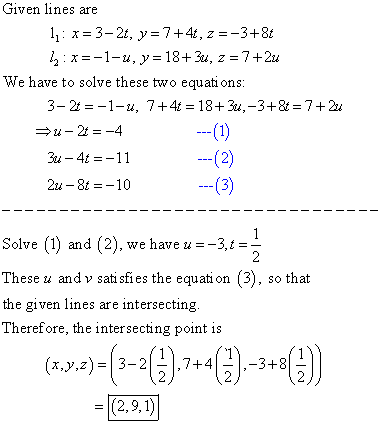# Determine whether the lines l1 and l2 are parallel, skew, or intersecting

Determine whether the lines l1 and l2 are parallel, skew, or intersecting. If they intersect, find the point of intersection.

l1: x=3-2t, y=7+4t, z=-3+8t
l2: x=-1-u, y=18+3u, z=7+2u